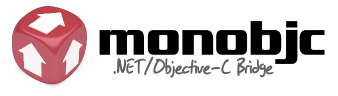﻿RotateByAngle Method (angle)Monobjc Documented Class LibraryRotateByAngle Method (angle)Namespaces ► Monobjc.AppKit ► NSView ► RotateByAngle(CGFloat)C#Visual BasicVisual C++

Rotates the receiver’s bounds rectangle by a specified degree value around the origin of the coordinate system, (0.0, 0.0).

Original signature is '- (void)rotateByAngle:(CGFloat)angle'

Available in Mac OS X v10.0 and later.Declaration Syntax
 C# Visual Basic Visual C++
```public virtual void RotateByAngle(
CGFloat angle
)```
```Public Overridable Sub RotateByAngle ( _
angle As CGFloat _
)```
```public:
virtual void RotateByAngle(
CGFloat angle
)```Parameters
angle (CGFloat)
A float value specifying the angle of rotation, in degrees.Version Information
• Available in Monobjc Bridge: 10.6 (For Mac OS X 10.6 and later), 10.5 (For Mac OS X 10.5 and later)

Assembly: Monobjc.AppKit (Module: Monobjc.AppKit)